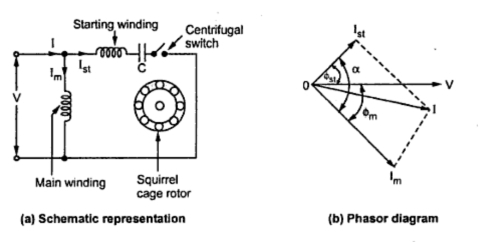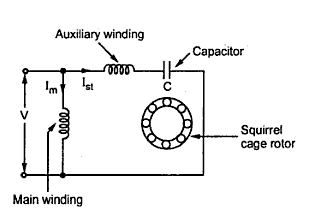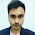### Capacitor Start Induction Motors

The construction of this type of motors is similar to the resistance split phase type. The difference is that in series with the auxiliary winding the capacitor is connected. The capacitive circuit draws a leading current, this feature used in this type to increase the split phase angle α between the two currents Im and Ist.
Depending upon whether capacitor remains in the circuit permanently or is disconnected from the circuit using centrifugal switch, these motors are classified as,
1. Capacitor start motor and       2. Capacitor start capacitor run motors
The connection of capacitor start motor is shown in the Fig. 1(a). The current Im lags the voltage by angle Φm while due to capacitor the current Ist leads the voltage by angleΦst. Hence there exists a large phase difference between the two currents which is almost 90o , which is an ideal case. The phasor diagram is shown in the Fig.1(b).Fig 1.  Capacitor start motor

The starting torque is proportional to 'α 'and hence such motors produce very high starting torque .
When speed approaches to 75 to 80% of the synchronous speed, the starting winding gets disconnected due to operation of the centrifugal switch. The capacitor remains in the circuit only at start hence it is called capacitor start motors.
Key point : In case of capacitor start capacitor run motor, there is no centrifugal switch and capacitor remain permanently in the circuit. This improves the power factor.
The schematic representation of such motor is shown in the Fig. 2.Fig. 2 Capacitor start capacitor run motor

The phasor diagram remains same as shown in the Fig.1(b). The performance not only at start but in running condition also depends on the capacitor C hence its value is to be designed so as to compromise between best starting and best running condition. Hence the starting torque available in such type of motor is about 50 to 100% of full load torque.
The direction of rotation, in both the types can be changed by interchanging the connection of main winding or auxiliary winding. The capacitor permanently in the circuit improves the power factor. These motors are more costly than split phase type motors.
The capacitor value can be selected as per the requirement of starting torque, the starting torque can be as high as 350 to 400 % of full load torque. The torque-speed characteristics is as shown in the Fig.3.Fig.3  Torque speed characteristic of capacitor split phase motor

Applications
These motors have high starting torque and hence are used for hard starting loads. These are used for compressors, conveyors, grinders, fans, blowers, refrigerators, air conditions etc. These are most commonly used motors. The capacitor start capacitor run motors are used in celling fans, blowers and air-circulations. These motors are available upto 6 kW.

Example : A 250 W, 230 V, 50 Hz capacitor start motor has the following impedances at standstill.
Main winding, Zm = 7 + j5 Ω
Auxiliary winding, Za = 11.5 + j5 Ω
Find the value of capacitor to be connected in series with the auxiliary winding to give a phase displacement of  between the currents in two windings. Draw the circuit and phasor diagram for motor.

Solution : Let Xc be the capacitive reactance to be connected with auxiliary winding at start, as shown in the Fig. 1(a).Fig. 1(a)

...              Za = 11.5 + j (5-Xc ) Ω
= 7 + j5 Ω = 8.6023
Now  Ia and Im must have a phase difference of 90o.  Im will lag the voltage by 35.5376o hence Ia must lead the voltage by (90o- 35.5376o ) i.e. 53.4624o , as shown in the Fig 1(b).Fig. 1(b)

The phase angle of  Za is,
Φa =tan-1((5 - Xc )/11.5) = -53.4624o
Key point :As leads, the phase angle of i.e. must be negative hence taken as
tan(-53.4624o ) = (5 - Xc )/11.5 i.e.
-1.34956 = (5 - Xc )/11.5
..                  Xc = 20.52 Ω = 1/(2πfC)
..                  C = 1/(2π x 50 x 20.52) = 155.1217 μF

1.what is the maximum starting torque by this method?

2.will the motor start if we replace the capacitor by an inductor of the same reactance?

3.What is power of 250 W. Is it power of motor or capacitor. If it is motor power this calculation is wrong. And the phase angle is not -53.4624, but -54.46.